# 小白学 Python 数据分析（16）：Matplotlib（一）坐标系

2019/04/10 10:10## 引言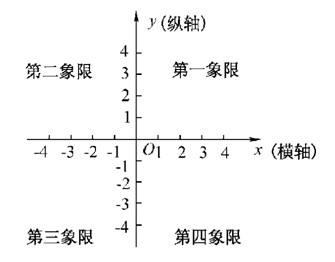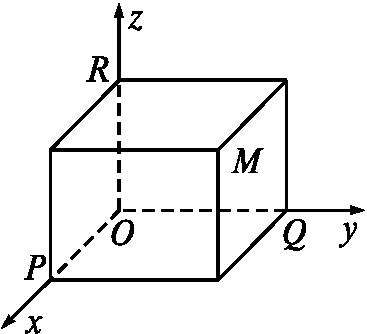## 画布

What？画布是啥？

emmmmmmmmmmm，这就类似于我们在电脑上画画一样，需要打开画图软件，创建一个空白的白板，这个白板就是我们后续画图的地方。import matplotlib.pyplot as plt

fig = plt.figure(figsize=(6, 6))


## 创建直角坐标系

### add_subplot

import matplotlib.pyplot as plt

fig = plt.figure(figsize=(6, 6))

fig.add_subplot(1,1,1)

plt.show()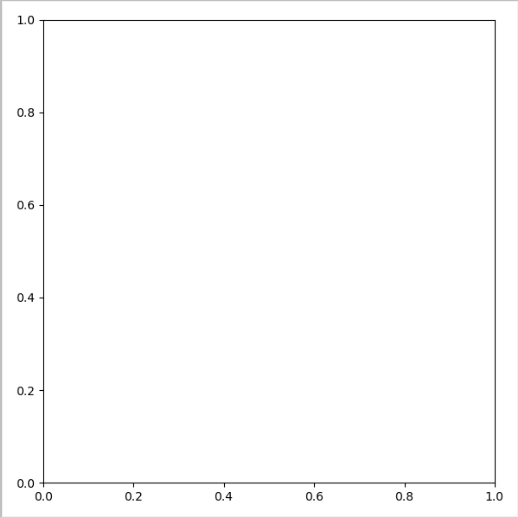import matplotlib.pyplot as plt

fig = plt.figure(figsize=(6, 6))

ax1 = fig.add_subplot(2,2,1)
ax2 = fig.add_subplot(2,2,2)
ax3 = fig.add_subplot(2,2,3)
ax4 = fig.add_subplot(2,2,4)

plt.show()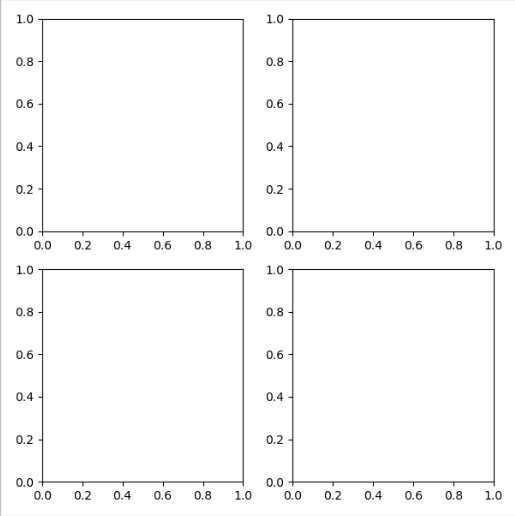ax1 = fig.add_subplot(221)


### subplot2grid

import matplotlib.pyplot as plt
import numpy as np

x = np.arange(4)
y = np.arange(4)

# 绘制折线图
plt.subplot2grid((2,2),(0,0))
plt.plot(x, y)

# 绘制柱状图
plt.subplot2grid((2,2),(0,1))
plt.bar(x, y)

plt.show()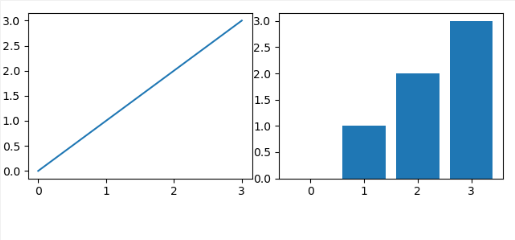### subplot

import matplotlib.pyplot as plt
import numpy as np

x = np.arange(4)
y = np.arange(4)

# 绘制折线图
plt.subplot(221)
plt.plot(x, y)

# 绘制柱状图
plt.subplot(222)
plt.bar(x, y)

plt.show()


### subplots

subplots 看起来和 subplot 很像，实际上也是非常像的，它和 subplot 的不同之处是 subplot 一次只能返回一个坐标系，而 subplots 一次可以返回多个坐标系。

import matplotlib.pyplot as plt
import numpy as np

x = np.arange(4)
y = np.arange(4)

fig, axes = plt.subplots(2, 2)
# 绘制折线图
axes[0,0].plot(x,y)
# 绘制柱状图
axes[0,1].bar(x,y)
plt.show()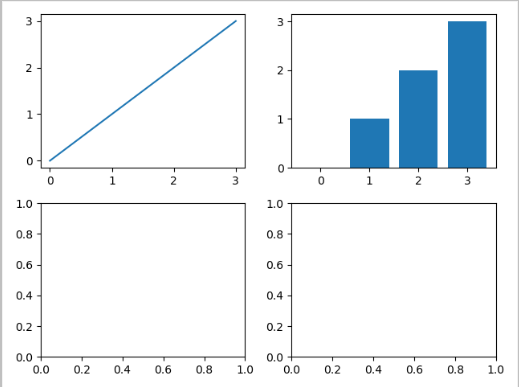## 小结

0
0 收藏

### 作者的其它热门文章0 评论
0 收藏
0